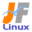Linux Kernel 2.6 Documentation: /usr/src/linux/Documentation/dell_rbu.txt

dell_rbu.txt

Dell rbu (Remote BIOS Update) \$B%I%i%\$%P\$N2r@b(B [\$B%W%l%\$%s%F%-%9%HHG(B]
• \$B86Cx:n
• \$BK]Lu.NS2mE5(B <zap03216@nifty.ne.jp>
• \$B%P!<%8%g%s(B: 2.6.29
• \$BK]LuF|;~(B: 2009/06/11
```

=========================================================
\$B\$3\$l\$O!"(B
Linux-2.6.29/Documentation/dell_rbu.txt \$B\$NOBLu(B
\$B\$G\$9!#(B
\$BK]LuCDBN!'(B JF \$B%W%m%8%'%/%H(B <http://www.linux.or.jp/JF/>
\$B99?7F|!'(B 2009/06/11
\$BK]Lu.NS2mE5(B <zap03216@nifty.ne.jp>
\$B9;@5http://support.dell.com register and you can find info on
# OpenManage and Dell Update packages (DUP).
# Libsmbios can also be used to update BIOS on Dell systems go to
# http://linux.dell.com/libsmbios/ for details.

\$B35MW(B:
\$B\$3\$N%I%i%\$%P\$r(B Dell OpenManage \$B\$b\$7\$/\$O(B Dell Update Packages \$B\$H\$N(B
\$BAH\$_9g\$o\$;\$GF0:n\$5\$;\$k\$H!"(BDell \$B\$N%5!<%P(B (1999\$BG/\$KH/Gd\$5\$l\$?\$b\$N0J9_(B)\$B!"(B
\$B%G%9%/%H%C%W%^%7%s\$d%N!<%H%Q%=%3%s(B (2005\$BG/\$KH/Gd\$5\$l\$?\$b\$N0J9_(B) \$B\$N(B
BIOS \$B\$,%"%C%W%G!<%H\$G\$-\$^\$9!#(B
http://support.dell.com \$B\$G%f!<%6!pJs\$,8+\$D\$+\$j\$^\$9!#(B
libsmbios \$B\$b(B Dell \$B\$N%7%9%F%`\$G(B BIOS \$B\$r%"%C%W%G!<%H\$9\$k\$N\$K;H\$(\$^\$9!#(B
\$B>\:Y\$O(B http://linux.dell.com/libsmbios/ \$B\$r8+\$F\$/\$@\$5\$\$!#(B

# Dell_RBU driver supports BIOS update using the monolithic image and packetized
# image methods. In case of monolithic the driver allocates a contiguous chunk
# of physical pages having the BIOS image. In case of packetized the app
# using the driver breaks the image in to packets of fixed sizes and the driver
# would place each packet in contiguous physical memory. The driver also
# If the dell_rbu driver is unloaded all the allocated memory is freed.

Dell_RBU \$B%I%i%\$%P\$O!"%b%N%j%7%C%/\$J%\$%a!<%8\$r;H\$&(B BIOS \$B%"%C%W%G!<%H\$H!"(B
\$B%Q%1%C%H2=\$5\$l\$?0lO"\$N%\$%a!<%872\$r;H\$&(B BIOS \$B%"%C%W%G!<%H\$r%5%]!<%H(B
\$B\$7\$F\$\$\$^\$9!#%b%N%j%7%C%/\$J%\$%a!<%8\$N>l9g!"%I%i%\$%P\$OO"B3\$7\$?J*M}%Z!<%8\$N(B
\$B%A%c%s%/(B(\$B2t(B)\$B\$r!"(BBIOS \$B%\$%a!<%8\$K3d\$jEv\$F\$^\$9!#%Q%1%C%H2=\$5\$l\$?(B
\$B%\$%a!<%872\$N>l9g!"%I%i%\$%P\$r;H\$&%"%W%j%1!<%7%g%s\$O%\$%a!<%8\$r(B
\$B8GDj%5%\$%:\$N%Q%1%C%H\$KJ,3d\$7!"\$=\$l\$+\$i%I%i%\$%P\$O3F%Q%1%C%H\$rO"B3\$7\$?(B
\$BJ*M}%a%b%j\$KCV\$-\$^\$9!#%I%i%\$%P\$O%Q%1%C%H\$rFI\$_La\$9\$?\$a\$K\$=\$l\$i\$N(B
\$B%j%s%/%j%9%H\$NJ];}\$b\$7\$F\$\$\$^\$9!#(B
dell_rbu \$B%I%i%\$%P\$,%"%s%m!<%I\$5\$l\$?>l9g!"3d\$jEv\$F\$i\$l\$?%a%b%j\$O\$9\$Y\$F(B
\$B2rJ|\$5\$l\$^\$9!#(B

# The rbu driver needs to have an application (as mentioned above)which will
# inform the BIOS to enable the update in the next system reboot.

rbu \$B%I%i%\$%P\$K\$O!"(B(\$B>e\$G?(\$l\$?(B) \$B%"%W%j%1!<%7%g%s\$,I,MW\$G\$9!#(B
\$B%"%W%j%1!<%7%g%s\$O!"l9g!"(BBIOS \$B%\$%a!<%8\$OJ*M}%a%b%jFb\$N\$R\$H\$D\$NO"B3\$7\$?%V%m%C%/\$K(B
\$B%3%T!<\$5\$l\$^\$9!#(B
\$B%Q%1%C%H%a%+%K%:%`\$N>l9g\$O!"C10l\$N%a%b%j\$,!"\$h\$j>.\$5\$JO"B3\$7\$?%a%b%j\$N(B
\$B%A%c%s%/(B(\$B2t(B)\$B\$KJ,3d\$5\$l\$k\$N\$G!"(BBIOS \$B%\$%a!<%8\$O\$3\$l\$i\$N%Q%1%C%H\$K(B
\$B;6:_\$7\$^\$9!#(B

# By default the driver uses monolithic memory for the update type. This can be
# changed to packets during the driver load time by specifying the load
# parameter image_type=packet.  This can also be changed later as below
# echo packet > /sys/devices/platform/dell_rbu/image_type

\$B%G%U%)%k%H\$G\$O!"%I%i%\$%P\$O%"%C%W%G!<%H\$N7A<0\$H\$7\$F%b%N%j%7%C%/%a%b%j\$r(B
\$B;H\$\$\$^\$9!#%I%i%\$%P\$N%m!<%I;~\$K%Q%i%a!<%?\$H\$7\$F(B image_type=packet \$B\$r(B
\$B;XDj\$9\$k\$H!"\$3\$N7A<0\$rJQ99\$G\$-\$^\$9!#l9g!"%Q%1%C%H\$,%a%b%j\$K%m!<%I\$5\$l\$kA0\$K(B
\$B%Q%1%C%H\$N%5%\$%:\$r;XDj\$7\$J\$/\$F\$O\$J\$j\$^\$;\$s!#8e\$KG[CV\$7\$^\$9!#\$3\$3\$G!"7k9g\$5\$l\$?%Q%1%C%H%X%C%@\$H(B
BIOS \$B%\$%a!<%8\$N2t\$r!";XDj\$5\$l\$?(B packet_size \$B\$K9g\$o\$;\$kI,MW\$,\$"\$j\$^\$9!#(B
\$B\$3\$&\$d\$C\$F\$R\$H\$D\$N%Q%1%C%H\$r:n\$j\$^\$9!#(B
\$BMxMQe\$NO"B3\$7\$?(B packet_size \$B\$N6u4V\$KCV\$\$\$F(B
\$B\$\$\$-\$^\$9!#(B
\$B\$3\$NJ}K!\$@\$H!"3Ne5-\$N%9%F%C%W(B2\$B\$rC<:\$7\$^\$9!#(B
\$B\$3\$l\$i\$N%(%s%H%j\$O!"

Linux \$B%+!<%M%k(B 2.6 \$BIUB0J8=q0lMw\$XLa\$k(B

JF Project \$B%a%\$%s%Z!<%8\$X(B
JF Project \$BA4HL\$K4X\$o\$k\$40U8+\$4MWK>\$O(B jf@listserv.linux.or.jp \$B\$^\$G\$*4j\$\$\$7\$^\$9(B.

```